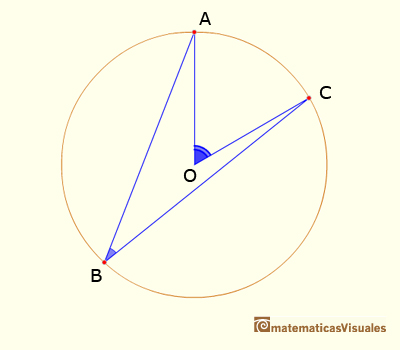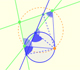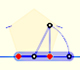Central and Inscribed Angle in a Circle: Case III

The general case of the Central Angle Theorem can be proved using the previous case. To do that we can draw the diameter that pases through the vertex of the inscribed angle. Then, depending on the position of the points, it is enough to add or to subtract some angles.Central angle in a circle is twice the angle inscribed in the circle.

In this position we have to add two central angles (and we use Case II):In this position we have to substract two central angles (and we use Case II):With Case I, Case II and this General Case we have finished the demostration of the Central Angle Theorem.REFERENCES

Euclides, The ElementsCentral angle in a circle is twice the angle inscribed in the circle.Using a ruler and a compass we can draw fifteen degrees angles. These are basic examples of the central and inscribed in a circle angles property.Each point in the circle circunscribed to a triangle give us a line (Wallace-Simson line)Interactive demonstration of the Wallace-Simson line.You can draw a regular pentagon given one of its sides constructing the golden ratio with ruler and compass.In his book 'Underweysung der Messung' Durer draw a non-regular pentagon with ruler and a fixed compass. It is a simple construction and a very good approximation of a regular pentagon.The diagonal of a regular pentagon are in golden ratio to its sides and the point of intersection of two diagonals of a regular pentagon are said to divide each other in the golden ratio or 'in extreme and mean ratio'.